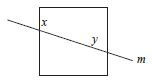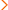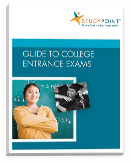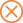Home > ed > Overview of the ACT Math Section

# Overview of the ACT Math Section

The ACT Math test consists of 60 questions that must be answered within the 60-minute time limit. All of the math questions are five-choice, multiple-choice questions. These questions draw from six areas of math that most students have covered by the end of their 11th grade year: pre-algebra, elementary algebra, intermediate algebra, coordinate geometry, plane geometry, and trigonometry. Below you can find more detailed information about the specific skills these questions test, as well as sample ACT Math questions and answer explanations.

ACT Math Test Question Types

Math Questions (Total of 60 questions)
Math Area Examples # of Questions
Pre-Algebra

whole numbers, fractions, decimals, and integers; positive integer powers and square roots; ratio, proportion, and percent; multiples and factors; absolute value; one variable, linear equations; probability and counting problems; data interpretation; and mean, median, and mode

14 questions

Elementary Algebra

variables, polynomials, factoring, quadratic equations, linear inequalities, integer exponents, and square roots

10 questions

Intermediate Algebra

quadratic formula, radical and rational expressions, inequalities, absolute value, sequences, systems of equations, quadratic inequalities, functions, matrices, polynomial roots, and complex numbers

9 questions

Coordinate Geometry

number line graphs; graphs of points, lines, polynomials, circles, and other curves; relationships between equations and graphs; slope; properties of parallel and perpendicular lines; distance formula; midpoint formula; transformations; and conics

9 questions

Plane Geometry

plane figures (triangles, rectangles, parallelograms, trapezoids, circles); angles; parallel lines; perpendicular lines; translations, reflections, and rotations; 3-D geometry; perimeter, area, and volume; and logical reasoning and proofs

14 questions

Trigonometry

right triangle trigonometric ratios; trigonometric functions, identities, and equations; and trigonometric functions modeling

4 questions

Sample ACT Math Test Questions

To give you a better feel for the format and content of the ACT Math test, let's take a look at a few sample ACT Math questions.

Pre-Algebra Question: Mean, Median, and Mode

70, 80, 50, 20, 80, 30, 80

Seven students took an art history exam. Their scores are listed above. Which of the following statements regarding the scores is true?

I. The average (arithmetic mean) is greater than 70.

II. The mode is greater than 70.

III. The median is greater than 70.

 A. None B. II only C. I and II only D. II and III only E. I, II, and III

Answer: First, find the average (arithmetic mean) of the test scores. Mean = (70 + 80 + 50 + 20 + 80 + 30 + 80)/7 = 58.57. 58.57 is not greater than 70. Therefore, item I. is not true, and we can eliminate choices C and E. Second, find the median of the test scores. To do so, put the test scores in ascending or descending order: 20, 30, 50, 70, 80, 80, 80. The median (the number in the middle) is 70. Therefore, item III. is not true, and we can eliminate choice D. Third, find the mode of the test scores (the number that appears most frequently): 80. Therefore, item II. is true. Choice B is the correct answer.

Plane Geometry Question: Plane Figures and Angles

If line m intersects the square as shown, what must the value of x + y be?A. 45 B. 75 C. 85 D. 90 E. 180

Answer: The sides of a square are parallel, so line m is a transversal to the sides of the square. That means that the angle next to (to the right of) angle y is equal to angle x by alternate interior angles. That also means that angle x is supplementary to angle y. Thus, x + y = 180. Choice E is the correct answer.

For additional ACT Math test sample questions, visit the ACT website.

Related Topics

LEARN MORE